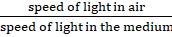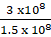### Solve the following examples. If the speed of light in a medium is 1.5 x 108 m/s, what is the absolute refractive index of the medium?

Question 7.

Solve the following examples.

If the speed of light in a medium is 1.5 x 108 m/s, what is the absolute refractive index of the medium?

Answer:

Absolute refractive index of medium is with respect to air.

So absolute refractive index of medium μ =Speed of light in air = 3 x 108 m/s

Speed of light in the given medium = 1.5 x 108 m/s

Absolute refractive index of medium μ =So μ = 2